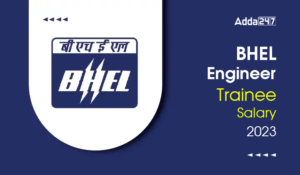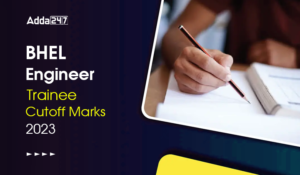Engineering Jobs   »   Mechincal Engineering quizs   »   UPPSC Lecturer Recruitment 2021, UPPSC Lecturer...

# UPPSC Lec’21 ME: Daily Practices Quiz. 13-Dec-2021

Quiz: Mechanical Engineering
Exam: UPPSC AE/Lecturer
Topic: RAC

Each question carries 3 marks
Negative marking: 1 mark
Time: 8 Minutes

Q1. A refrigerating machine working on reversed Carnot cycle takes out 2 kW per minute of heat from the system while between temperature limits of 300 K and 200 K. COP and power consumed by the cycle will be respectively.
(a) 1 and 1 kW
(b) 1 and 2 kW
(c) 2 and 1 kW
(d) 2 and 2 kW

Q2. Match list I with list II and select the correct answer using the codes given below the lists:
List – I List – II
A. Bell Coleman refrigeration 1. Compressor
B. Vapour compression refrigeration 2. Generator
C. Absorption refrigeration 3. Flash chamber
D. Jet refrigeration 4. Expansion cylinder
codes
A B V D
(a) 1 4 3 2
(b) 4 1 3 2
(c) 1 4 2 3
(d) 4 1 2 3

Q3. What is an azeotrope?
(a) A non-halogenic refrigerant
(b) A refrigerant dissolved in alcohol
(c) A mixture of refrigerants without phase separation
(d) An eco-friendly refrigerant

Q4. Air at 35°C DBT and 25°C dew point temperature passes through the water shower whose temperature is maintained at 20°C. What is the process involved?
(a) cooling and humidification
(b) sensible cooling
(c) cooling and dehumidification
(d) heating and humidification

Q5. For an air-conditioned space, RTH = 100 kW, RSHF = 0.75, volume flow rate = 100 m³/min, and indoor design specific humidity is 0.01 kg/kg of dry air. What is the specific humidity of the supply air?
(a) 0.010
(b) 0.0075
(c) 0.005
(d) 0.0025

Q6. In an air-standard Diesel cycle; r is the compression ratio, ρ is the fuel cut-off ratio and γ is the adiabatic index (C_p/C_V ). Its air standard efficiency is given by
(a) η=1-{1/(γ.r^(γ-1) ).((ρ^γ-1))/((ρ-1))}
(b) η=1-{1/(γ.r^(γ-1) ).((ρ^(γ-1)-1))/((ρ-1))}
(c) η=1-{1/(γ.r^(γ-1) ).((ρ^(γ-1) ))/((ρ-1))}
(d) η=1-{1/(γ.r^γ ).((ρ^(γ-1)-1))/((ρ-1))}

Solutions

S1. Ans.(c)
Sol. Given data

Q_2=2 kW
T_1=300 K
T_2 = 200 K
COP=T_2/(T_1-T_2 )
=200/(300-200)=2
Also,
COP=Q_2/W
∴2=2/W
or,W=2/2=1 kW per minute

S2. Ans.(d)
Sol.
1. Bell Coleman refrigeration: Expansion cylinder
2. Vapour compression refrigeration: compressor
3. Absorption refrigeration: Generator
4. Jet Refrigeration: Flash chamber

S3. Ans.(c)
Sol. Azeotrope is a mixture of refrigerants without phase separation.

S4. Ans.(c)
Sol. As the temperature of water shower is less than dew point temperature hence, water vapour starts condensing. Thus, process involved is cooling and dehumidification.

S5. Ans.(c)
Sol.
RSHF=RSH/RTH
∴RSH=0.75×100=75 kW
RLH=100-75=25 kW
RLH = 50 cmm (w1 – w2)
W2 = 0.005 kg/kg of dry air

S6. Ans.(a)
Sol.
η_diesel=1-{1/r^(γ-1) (ρ^γ-1)/(γ(ρ-1)}
η of diesel engine increases as the load on the engine decreases and at limiting condition becomes to Otto. With increase in load, cut off ratio increases.

Sharing is caring!

•GATE 2023 Admit Card Out, Direct Link fo...
•BHEL Engineer Trainee Salary 2023, Perks...
•BHEL Engineer Trainee Cutoff Marks 2023,...
•UPSC ESE Admit Card 2023, Download Preli...
•Last Week Preparation Strategy for GATE ...
•BARC OCES Syllabus 2023 Checkout Latest ...
•SAMEER Recruitment 2023 Out For 35 Posts...